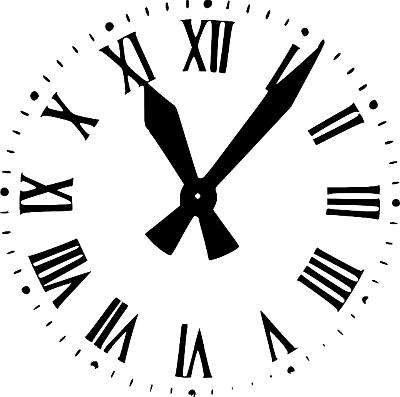# Minutes to Hours Calculator

Instructions: Use this calculator to convert minutes into hours, showing all the steps of the conversion. For example, if you want to convert 55.4 minutes into hours, please type in "55.4" in the form below:Type the number of minutes M you want to convert (Ex: 55.45, etc)

## Minutes to Hours Calculator

Time can be measured in different ways, and for that purpose, we have different units of time. Hours and minutes are very commonly used times units that are also commonly converted between each other.

Why would we have many units of time? Because it is practical, depending on the situation. For example, supposed you are at the doctor's office lobby, and you ask the front desk person, "how long until the doctor can see me?", and the person responds "0.000028539 years".

That answer could be perfectly valid, because "0.000028539 years" is the same as 15 minutes. But you would perhaps question the mental sanity of anyone who would respond like that.

## How do you convert minutes into hours?

You need to start with the basic conversion of 60 minutes = 1 hour. From there, using direct proportionality, you can compute the following basic hours to minutes formula

$M = 60 H$

which states that when you have H hours, you will have will have $$60 \times H = 60 H$$ minutes. And then we can solve for H, so we get:

$H = \displaystyle \frac{M}{60}$

which corresponds to the formula to convert minutes into hours.

Step 1: Identify clearly the number of minutes you want to convert, and call it M. Make sure is measured in minutes

Step 2: The way you change minutes to hours is by using the following formula:

$H = \displaystyle \frac{M}{60}$

Step 3: Plugging the value of M (number of minutes) into the above formula, you get the corresponding number of hours

### How to convert minutes to hours in Excel?

Super easy. You just type "A1/60" or "A1*1/60" in a cell, assuming that A1 is a cell that contains the number of minutes you are looking to convert.### Summary: How to go around to convert minutes to hours?

Super simple. Get the number of minutes M you want to convert, and divide them by 60. That is it.

### Example: Conversion of Seconds to Hours

Question: Convert 48 minutes into hours.

Solution:

We know that we need to get the number of minutes and divide them by 60. We find that :

$H = \displaystyle \frac{M}{60} = \displaystyle \frac{48}{60} = 0.8$

Then, using the minutes to hours conversion formula leads to 48 minutes = 0.8 hours. Don't forget to CLEARLY specify units!

### Example 2

Question Convert 1500 minutes to hours

Solution:

We need to establish the amount in minutes that we need to convert. Here, we need to convert 1500 minutes. We use the following formula

$H = \displaystyle \frac{M}{60} = \displaystyle \frac{1500}{60} = 25$

Hence, by applying the conversion formula, we get that 1500 minutes = 25 hours (a bit more than a day).

### Other fine Calculators relating hours, minutes, and seconds?

Most time unit conversions are easy if you rely on the basic proportionality formula. But sometimes you may forget. To go the other way around, you can use this hours to minutes calculator, for example, when an event your are measuring the time is too short, and using hours brings small decimal numbers, which would be more prone to errors.

A larger leap for which you can use some help is the conversion from hours to seconds and seconds to hours. There are obvious practical uses for both.